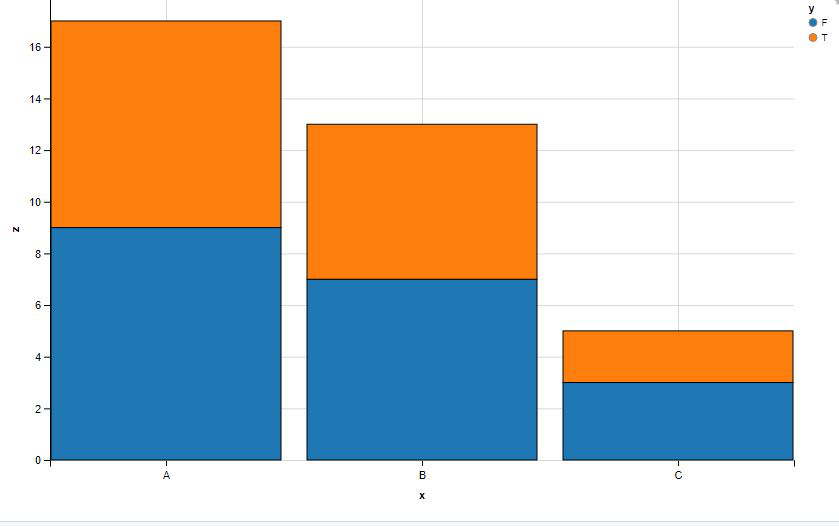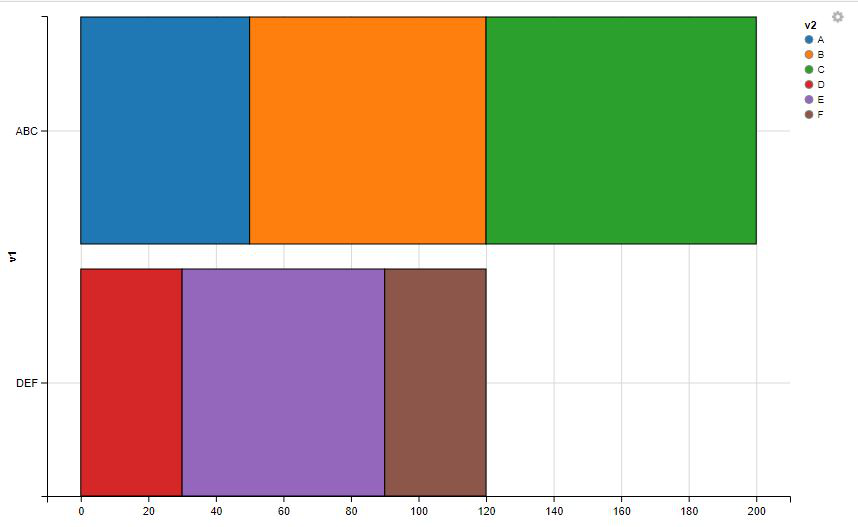Open in App
Not now

# How to create stacked bar chart using ggvis in R

• Last Updated : 09 Sep, 2022

In this article, we will be looking at the approach for creating a stacked bar chart using ggvis in the R programming language.

## Create the stacked bar chart with the layer_bars function of ggvis package

In this approach to create the stacked bar chart with layer_bars function from the ggvis package, the user first needs to install and import the ggvis package to the working R console, here the ggvis package is responsive to provide the functionality to create the stacked bar chart, further, the user needs to call the layer_bars() function with the specific syntax as showing in the examples used passed with the stack argument set to true and pass the required parameters accordingly as per the user requirement as this will be leading to the plotting of the stacked bar chart in the R programming language.

Syntax to install and import the ggvis package in the working console:

```install.packages('ggvis')
library(ggvis)```

### layer_bars function:

This will add bars to a plot. The exact behavior is complicated because the term bar chart is used to describe four important variations on a theme.

The action of layer_bars depends on two factors: whether or not a y prop has been specified, and whether the x props is continuous or categorical.

Syntax: layer_bars(vis, …, stack = TRUE, width = NULL)

Parameters:

• vis: Visualization to modify
• …: Visual properties used to override defaults.
• stack: If there are multiple bars to be drawn at an x location.
• width: Width of each bar.

Example 1:

In this example, we have created a data frame with three rows and 6 columns, then with the call of the layer_bars() function from the ggvis function passed with the data frame in the specific syntax given to create the stacked bar chart in the R programming language.

## R

 `# Import the required libraries` `library``(ggvis)`   `# Create Data` `x<-``c``(``"A"``,``"B"``,``"C"``,``"A"``,``"B"``,``"C"``)` `y<-``c``(``"T"``,``"F"``,``"T"``,``"F"``,``"T"``,``"F"``)` `z<-``c``(8,7,2,9,6,3)` `df<-``data.frame``(x,y,z)`   `# Create the stacked bar` `# chart with the layer_bars function ` `df %>% ``ggvis``(x=~x, y=~z, fill=~y) %>% ` `group_by``(y) %>%``layer_bars``()`

Output:Example 2:

In this example, we have created the data frame of 3 rows and 6 columns, then further called the compute_stack and the layers_reacts function from the ggvis package passed with the required parameters and given in the required syntax to get the bar stacked chart in the vertical direction in the R programming language,

## R

 `# Import the required libraries` `library``(ggvis)`   `# Create Data` `df<-``data.frame``(v1    = ``c``(``"ABC"``, ``"ABC"``, ``"ABC"``,` `                         ``"DEF"``,``"DEF"``,``"DEF"``), ` `               ``v2    = ``c``(``"A"`  `, ``"B"`  `, ``"C"`  `,` `                         ``"D"`  `,``"E"`  `,``"F"`  `),` `               ``value = ``c``(50   ,  70  ,  80   ,` `                         ``30  , 60  , 30  ))`   `# Create the stacked bar ` `# chart with the layer_bars function ` `df %>%  ``ggvis``(y = ~v1, fill = ~v2) %>%` `  ``compute_stack``(stack_var = ~value,` `                ``group_var = ~ v1) %>%` `  ``layer_rects``(x = ~stack_lwr_,` `              ``x2 = ~stack_upr_, ` `              ``height = ``band``())`

Output:My Personal Notes arrow_drop_up
Related Articles